# Improved Behavior of Auto Tolerance in R2017a

Are you familiar with the meaning of auto for the Absolute tolerance of Simulink variable-step solvers?

In R2017a, we decided to change the meaning of auto for the Absolute Tolerance... If your model is using this setting, I recommend you keep reading to see if and how you might be affected.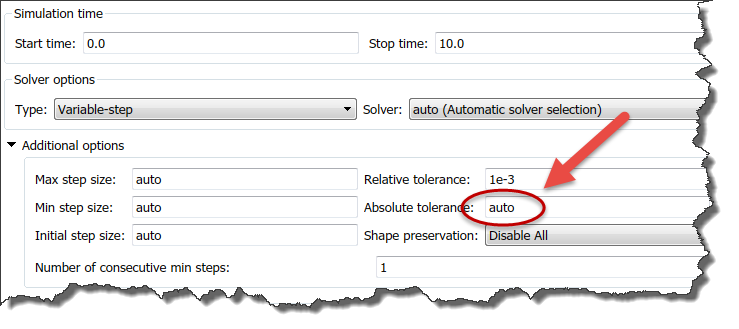How Tolerances work?

To learn more about how error tolerancing works for Simulink variable-step solvers, I recommend going through this documentation page.

What you will find is that for each continuous state in a model, an error is computed. This error is computed differently for each solver, but in general variable-steps solver integrate continuous states using two methods, and the error is the difference between both.

If we take the simplest of our variable-step solvers, ode23, it computes its output y(n+1) using a third-order approximation and also computes a second-order approximation z(n+1) so the difference can be used for error control and adapting the step size.To see that in action, I recommend using the Simulink command-line debugger, in particular a combination of strace 4, trace gcb, and step top.

Once the error is computed for each state, we ensure that the error is smaller than the absolute tolerance, or the relative tolerance multiplied by the state amplitude, whichever one is the largest.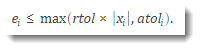Over time, this can look like this: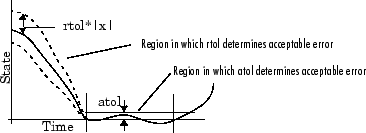What's new in R2017a?

Before R2017a, when choosing a value of auto for the absolute tolerance, the initial value used was systematically 1e-6. Then if the amplitude of the states increases during the simulation, the absolute tolerance would also increase to a value corresponding to the state value multiplied by the relative tolerance.

In R2017a, the meaning of auto for absolute tolerance is changed to the value of the relative tolerance multiplied by 0.001. We believe that this new definition of auto reduces the chances of getting inaccurate results.

To illustrate the difference, let's take this simple model implementing a first order transfer function:In R2016b, if I set the relative tolerance to 1e-6 and leave the absolute tolerance to auto, the results look like: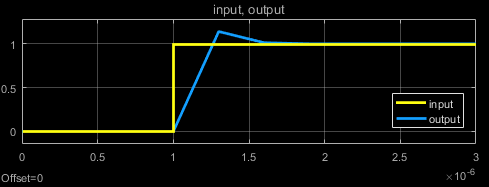As you can see, this result is far from accurate, a first order transfer function should not overshoot like that. The problem is that the value of the state is smaller than 1e-6, and the absolute tolerance decided by "auto" is 1e-6. With such setting, pretty much any answer would be within tolerances and considered valid.

In R2017a, when I simulate this model the results I get is: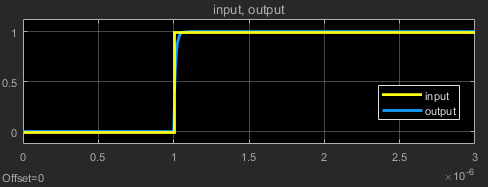With the new definition of "auto", the absolute tolerance used is now relTol*0.001 = 1e-9, giving the expected answer.

Consequences?

In R2016b and prior releases, you might have ended up setting the relative tolerance to a value much smaller than 1e-3, while leaving the absolute tolerance to auto. This would have compensated for the lack of accuracy due to the auto absolute tolerance being always larger than 1e-6.

In R2017a, the lower bound on auto absolute tolerance is no longer fixed at 1e-6. In case this tighter tolerance slows down your simulation, the first thing I would recommend is leaving the absolute tolerance to auto, and try increasing the relative tolerance close to its default value of 1e-3 (or 1e-4) to get results of comparable accuracy.

You may also want to try different values for relative and absolute tolerances and see what gives the best trade-off between performance and accuracy for your model.

Now it's your turn

Let us know in the comments below if you are impacted by this change, we would like to hear.

|

### Comments

To leave a comment, please click here to sign in to your MathWorks Account or create a new one.